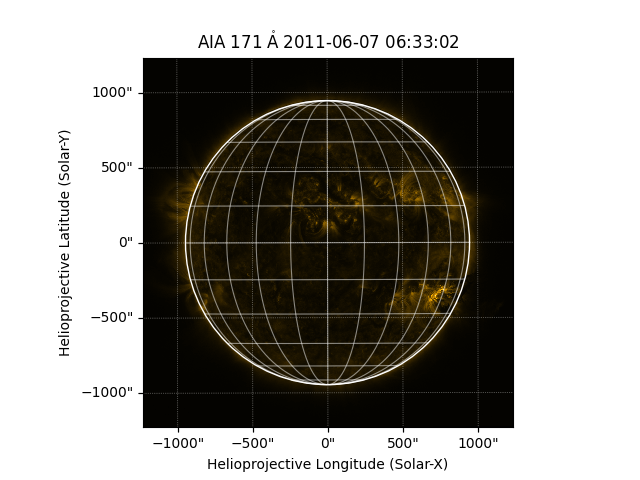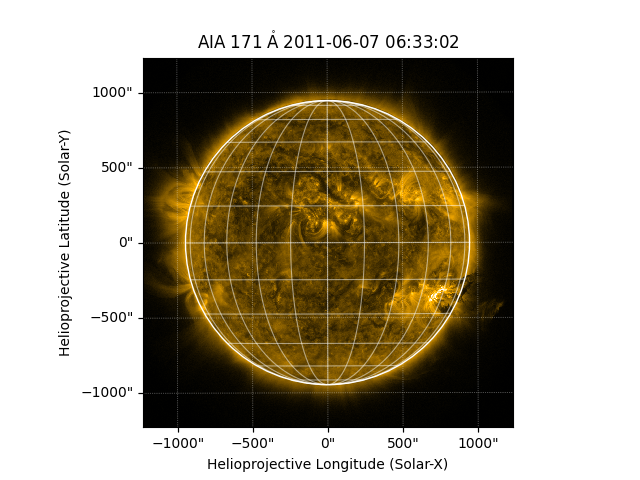# Plotting a map#

How to create a plot of a map.

import matplotlib.pyplot as plt

import astropy.units as u

import sunpy.map
from sunpy.data.sample import AIA_171_IMAGE


aiamap = sunpy.map.Map(AIA_171_IMAGE)


Let’s plot the result. Setting the projection is necessary to ensure that pixels can be converted accurately to coordinates values.

fig = plt.figure()
aiamap.plot(axes=ax)
aiamap.draw_limb(axes=ax)
aiamap.draw_grid(axes=ax)
plt.show()The above image looks “dark” because the color scale is accounting for the small set of pixels that are extremely bright. We can use the keyword clip_interval to clip out pixels with extreme values. Here, we clip out the darkest 1% of pixels and the brightest 0.01% of pixels.

fig = plt.figure()